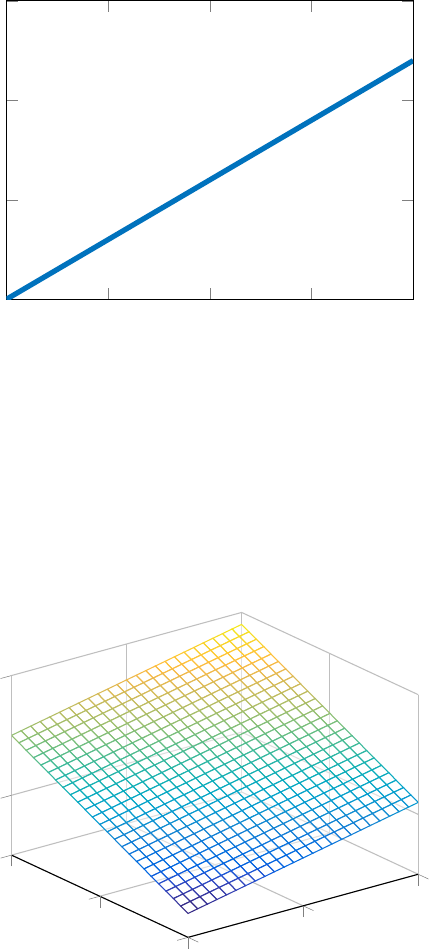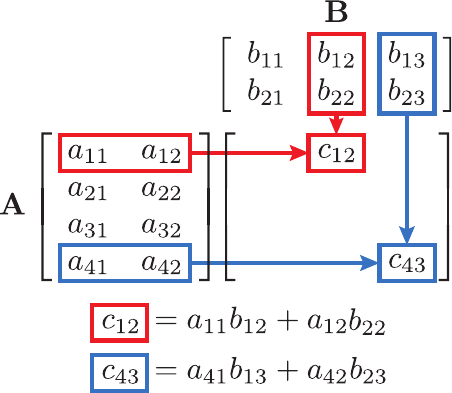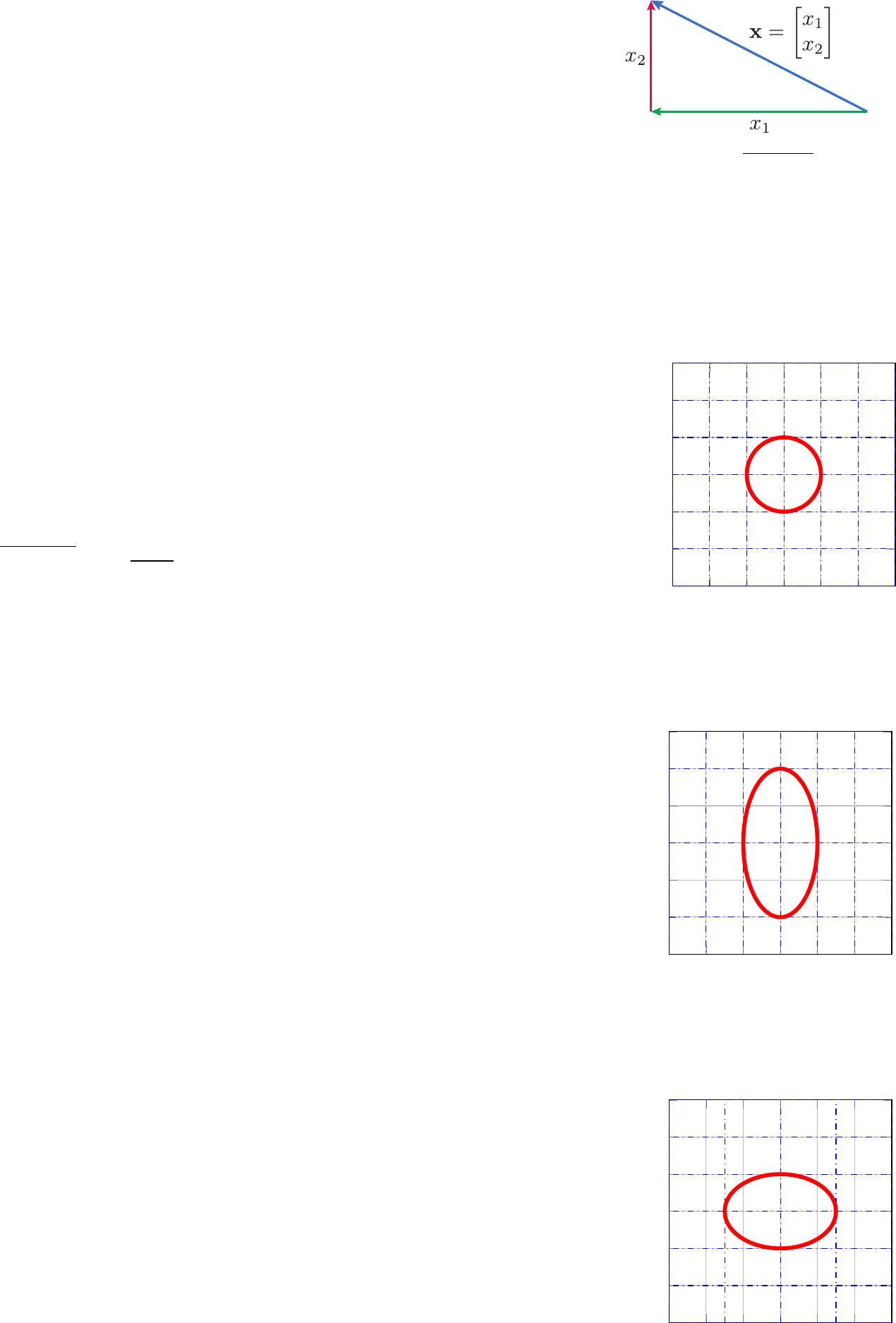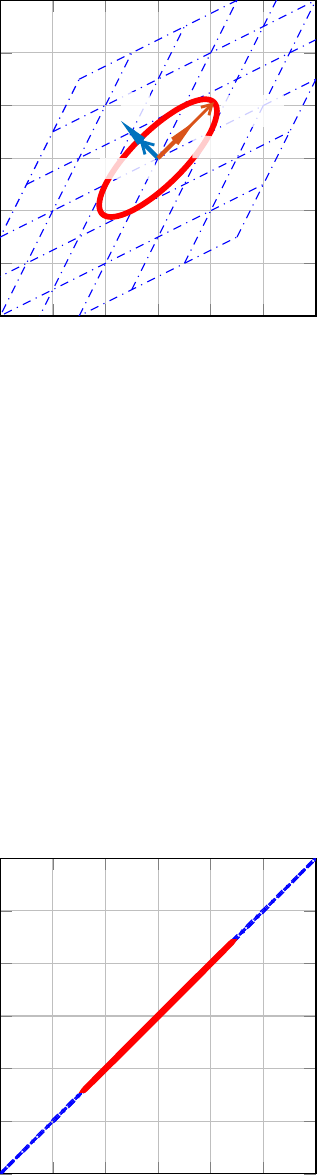2
Linear Algebra
Linear algebra is employed in a majority of machine learning meth-
ods and algorithms. Before going further, it is essential to under-
stand the mathematical notation and basic operations.
2.1 Notation
We employ lowercase letters
x, s, v, ···
in order to describe variables
x :scalarvariable
x :columnvector
X :matrix
x
i
[x]
i
: i
th
element of a vector
x
ij
[X]
ij
: {i, j}
th
element of a
matrix
that can lie in speciﬁc domains such as real numbers
R
, real posi-
tive
R
+
, integers
Z
, closed intervals [
·, ·
], open intervals (
·, ·
), and so
on. Often, the problems studied involve multiple variables that can Examples of variables
x
belonging to
dierent domains
x 2 R (1, 1)
2 R
+
(0, 1)
2 Z {···, 1, 0, 1, 2, ···}
be regrouped in arrays. A 1-D array or vector contai n in g sc alar s i s
represented as
x =
2
6
6
6
4
x
1
x
2
.
.
.
x
n
3
7
7
7
5
.
By convention, a vector
x
implicitly refers to a
n
1 column vector.
For exampl e, if each element
x
i
[
x
]
i
is a real number [
x
]
i
2 R
for
all
i
from 1 to
n
, then the vector belongs to the
n
-dimensional real
domain
R
n
. This last state me nt can be expressed mathematically as
[
x
]
i
2 R, 8i 2{
1:
n}!x 2 R
n
. In mach i ne learning, it is common
to have 2-D arrays or matrices,
X =
2
6
6
6
4
x
11
x
12
··· x
1n
x
21
x
22
··· x
2n
.
.
.
.
.
.
.
.
.
.
.
.
x
m1
x
m2
··· x
mn
3
7
7
7
5
,
where, for example, if each
x
ij
[
X
]
ij
2 R, 8i 2{
1:
m},j 2{
1:
n}!X 2 R
mn
. Arrays beyond two dimensi ons are referred to as
tensors. Although tensors are widely employed in the ﬁeld of neural
networks, they will not be treated in this book.j.-a. goulet 10
There are several matrices with speci ﬁ c properties: A diagonal
matrix is square and has only terms on its mai n diagonal,
Y = diag(x)=
2
6
6
6
4
x
1
0 ··· 0
0 x
2
··· 0
.
.
.
.
.
.
.
.
.
.
.
.
00··· x
n
3
7
7
7
5
nn
.
An identity matrix
I
is similar to a diagonal matrix except that
element s on the main diagonal are 1, and 0 everywhere else,
I =
2
6
6
6
4
10··· 0
01··· 0
.
.
.
.
.
.
.
.
.
.
.
.
00··· 1
3
7
7
7
5
nn
.
A block diagonal matrix concatenates several matrices on t h e m ai n
A =
1 2
3 4
, B =
2
4
4 5 6
7 8 9
10 11 12
3
5
.
blkdiag(A, B)=
2
6
6
6
6
4
1 2 000
3 4 000
00 4 5 6
00 7 8 9
0010 11 12
3
7
7
7
7
5
diagonal of a sin gle matrix,
blkdiag(A, B)=
A0
0B
.
We can mani pu l at e t h e di me ns ion s of matrices using the transpo-
sition oper ati on so that indices are permuted [
X
|
]
ij
=[
X
]
ji
. For
example,
X =
x
11
x
12
x
13
x
21
x
22
x
23
! X
|
=
2
4
x
11
x
21
x
12
x
22
x
13
x
23
3
5
.
The trace of a square matrix
X
corresponds to the sum of the
element s on its main diagonal,
tr(X)=
n
X
i=1
x
ii
.
2.2 Operations
2 1 0 1 2
5
0
5
10
x
y
y =3x +1
= ax + b
a =3,b=1
Figure 2.1: 1-D plot representing a linear
system, y = ax + b.
2
0
2
2
0
2
0
10
x
1
x
2
y
y = x
1
+2x
2
+3
= a
|
x + b
a =
1
2
, x =
x
1
x
2
,b=3
Figure 2.2: 2-D plot representing a linear
system, y = a
|
x + b.
In the context of machine learning, linear algebra is employed
because of its capacity to model linear sys tem s of equations in a
format that is compact and well suite d for computer calculations.
In a 1-D case, such as the one represented in ﬁgure 2.1, the
x
space
is mapped into the
y
space,
R ! R
, through a linear (i.e., ane)
function. Figure 2.2 presents an ex amp l e of a 2-D linear function
where the
x
space is mapped into the
y
space,
R
2
! R
. This can
be generalized to linear systems
y
=
Ax
+
b
, deﬁning a mapping
so that
R
n
! R
m
,where
x
and
y
are respectively
n
1 and
m
1probabilistic machine learning for civil engineers 11
vect or s. The product of the matrix
A
with the vector
x
is deﬁned
as [Ax]
i
=
P
j
[A]
ij
· [x]
j
.
In more general cases, linear algebra is employed to multiply a
matrix
A
of size
n k
with another matrix
B
of size
k m
,sothe
result is a n m matr ix ,
C = AB
= A B.
The matrix multiplication operation f ol l ows
[C]
ij
=
P
k
[
A
]
ik
·
[
B
]
kj
,
as illustrated in ﬁgure 2.3. Following the requirement on the size of
the matrices multiplied, this operation is not generally commutative
so that
AB 6
=
BA
. Matrix multiplication follows several pr operties
such as the following:
Distributivity A(B + C)=AB + AC
Associativity A(BC)=(AB)C
Conjugate transposability (AB)
|
= B
|
A
|
.
Figure 2.3: Example of matrix multiplica-
tion operation C = AB.
When the matrix multiplication operator is applied to
n
1 vectors,
it reduces to the inner product,
x
|
y x · y
=[x
1
··· x
n
]
2
6
4
y
1
.
.
.
y
n
3
7
5
=
P
n
i=1
x
i
y
i
.
Another common operation is the Hadamar product or element-
wise product, which is represented by the symbol
. It consists in
mult i pl y i ng each term from matrices
A
mn
and
B
mn
in order to
obtain C
mn
,
C = A B
[C]
ij
=[A]
ij
· [B]
ij
.
The element-wise product is seldom em pl oyed to deﬁn e m ath em at i-
cal equations; however, it is extensively employed when implement-
ing these equations in a computer language. Matrix additi on i s by
deﬁnition an element-wise operation that applies only to matrices of
same dimensions,
C = A + B
[C]
ij
=[A]
ij
+[B]
ij
.
One last key operation is the matri x invers i on
A
1
. In order to
be invertible, a matrix must be square and must n ot have linearly
dependent rows or columns. The product of a matrix with its in-
Linearly dep endent vectors
Vectors
x
1
2 R
n
and
x
2
2 R
n
are linearly
dependent if a nonzero vector
y 2 R
2
exists, such that y
1
x
1
+ y
2
x
2
= 0.j.-a. goulet 12
vers e i s e qu al to the identity matrix
A
1
A
=
I
. Matrix inversion is
particularly useful for solving linear s ys t ems of equations,
Ax = b,
A
1
Ax = A
1
b,
Ix = A
1
b,
x = A
1
b.
||x||
2
=
q
x
2
1
+ x
2
2
||x||
1
= |x
1
| + |x
2
|
||x||
1
=max|x
1
,x
2
| = |x
1
|
Figure 2.4: Examples of applications of
dierent norms for computing the length of
avectorx.
2.3 Norms
Norms measure how large a vector is. In a generic way, the
L
p
-norm
is deﬁned as
||x||
p
=
X
i
|[x]
i
|
p
!
1/p
.
Special cases of inte re st are
||x||
2
=
s
X
i
[x]
2
i
p
x
|
x (Euclidian norm)
||x||
1
=
X
i
|[x]
i
| (Manhattan norm)
||x||
1
= max
i
|[x]
i
|. (Max norm)
These cases are illustrated in ﬁgure 2.4. Among all cases, the
L
2
-
norm (Euclidian distance) is the most common. For example,
§
8.1.1 presents for the context of linear regression how choosing a
Euclidian norm to measure the distance between observations and
model predictions allows solving the parameter estimation problem
analytically.
2.4 Transformations
Machi ne learning involves transformations from one space to an-
other. In the context of linear algebr a, we are i nterested in the
special case of linear transformations.
2.4.1 Linear Transformations
3 2 1 0 1 2 3
3
2
1
0
1
2
3
x
0
1
x
0
2
(a) A =
10
01
, det(A)=1
3 2 1 0 1 2 3
3
2
1
0
1
2
3
x
0
1
x
0
2
(b) A =
10
02
, det(A)=2
3 2 1 0 1 2 3
3
2
1
0
1
2
3
x
0
1
x
0
2
(c) A =
1.50
01
, det(A)=1.5
Figure 2.5: Examples of linear transforma-
tions x
0
= Ax.
Figure 2.1 presented an example for a
R ! R
linear transforma-
tion. More generally, a
n n
square matrix can be employed to
perform a
R
n
! R
n
linear transformation through multiplication.
Figures 2.5a–c illustrate how a matrix
A
transforms a space
x
into
another
x
0
using the matrix product operation
x
0
=
Ax
.Thede-
formation of the circle and the underlying grid (see (a) ) show the
eect of various transformations. Note that the terms on the mainprobabilistic machine learning for civil engineers 13
diagonal of
A
contr ol the transformations along the
x
0
1
and
x
0
2
axes,
and the nondiagonal terms control the t ran sf or mat i on dependency
between both axes, (s ee, for example, ﬁ gu re 2.6).
The determinant of a square mat r ix
A
measures how much the
transformation contracts or expands the space:
det(A) = 1: preserves the space/volume
det
(
A
) = 0: collapses the spac e/volume along a subset of dimen-
sions, for example, 2-D space ! 1-D space (see ﬁgu re 2.7)
In the examples presented in ﬁgure 2.5a–c, the determinant quan-
tiﬁes how much the area/volume is changed in the transformed
space; for the circle, it corresponds to the change of area caused by
the transformation. As shown in ﬁgure 2. 5a, if
A
=
I
, the transfor-
mation has no eect so
det
(
A
) = 1. For a square matrix [
A
]
nn
,
det(A):R
nn
! R.
2.4.2 Eigen Decomposition
Linear transformations operate on several dimensions, such as in
the case presented in ﬁgure 2.6 where the transformation introduces
dependency between variables. Eigen decomposition enables ﬁnding
a linear transformation that removes the dependency while preserv-
ing the area/volume. A square matrix [
A
]
nn
can be decomposed
in eigenvectors
{
1
, ··· ,
n
}
and eigenvalues
{
1
, ··· ,
n
}
.Inits
matrix form,
A = Vdiag()V
1
,
where
V =[
1
···
n
]
=[
1
···
n
]
|
.
Figure 2.6 presents the eigen decompos it i on of the transformation
x
0
=
Ax
. Eigenvectors
1
and
2
describe the new referential into
which the transformation is indepe nd ently applied to each axis.
Eigenvalues
1
and
2
describe the transformation magnitude along
each eigenvector.
3 2 1 0 1 2 3
3
2
1
0
1
2
3
2
1
2
2
1
1
x
0
1
x
0
2
x
0
= Ax
A =
10.5
0.51
V =[
1
2
]=
0.71 0.71
0.71 0.71
=[0.51.5]
|
Figure 2.6: Example of eigen decomposi-
tion, A = Vdiag()V
1
.
3 2 1 0 1 2 3
3
2
1
0
1
2
3
x
0
1
x
0
2
A =
10.99
0.99 1
, det(A)=0.02
Figure 2.7: Example of a nearly singular
transformation.
A matrix is positive deﬁnite if all eigenvalues
>
0, and a matrix
is positive semideﬁnite (PSD) if all eigenvalues
0. The deter-
minant of a matrix corresponds to the pr oduct of its eigenvalues.
Therefore, in the case where one eigenvalue equals zero, it indicates
that two or more dimensions are linearly dependent and have col-
lapsed into a single one. The transformat i on matrix is then said
to b e si ngu lar . Figure 2.7 presents an examp l e of a nearly singular
transformation. For a positive semideﬁnite mat ri x
A
and for any
j.-a. goulet 14
vector x, the foll owing relation holds:
x
|
Ax 0.
This property is employed in
§
3.3.5 to deﬁne the requirements for
an admissible covariance matri x .
A more exhaustive review of linear al geb ra can be found in
dedicated textbooks su ch as the one by Kreyszig.
1
1